# Python Program to Remove Word from Sentence

This article is created to cover some programs in Python, that removes a particular word (entered by user) from a string (entered by user). Here are the list of approaches used to do the task:

• Remove a Word from String using replace()
• Using List

## Remove a Word from String using replace()

To remove or delete a desired word from a given sentence or string in Python, you have to ask from the user to enter the string and then ask to enter the word present in the string to delete all the occurrence of that word from the string and print the new string like shown in the program given below:

```print("Enter the String: ")
text = input()

print("Enter a Word to Delete: ")
word = input()

text = text.replace(word, "")

print()
print(text)```

Here is the initial output produced by this Python program: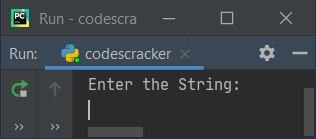Now supply the input say welcome to codescracker as string, then to as word to delete. Here is the sample output with exactly same input: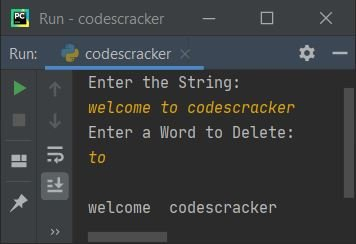From above program, the following code (statement):

`text = text.replace(word, "")`

states that the value of word gets replaced with "" (nothing) in given string stored in text. That is, in place of every word's value, nothing ("") gets placed. In this way, the word gets deleted or removed

#### Modified Version of Previous Program

The end used in this program, to skip inserting an automatic newline using print(). The + is used to concatenate string. And the \" is used to print " on output:

```print("Enter String: ", end="")
text = input()

print("Enter a Word to Delete: ", end="")
word = input()

wordlist = text.split()
if word in wordlist:
text = text.replace(word, "\b")
print("\nNew String without \"" +word+ "\":")
print(text)
else:

Here is its sample run with string input as this is python programming, then word input as python: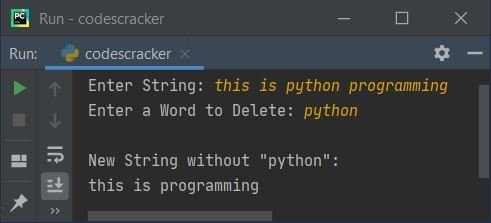Here is another sample run with same string input, but word input as codescracker:Note - The split() method splits the string into words.

For example, if the string stored in text are this is python programming, then after executing the following code:

`wordlist = text.split()`

the list named wordlist is created with its values (elements) as:

`['this', 'is', 'python', 'programming']`

Note - The "\b" is used to insert backspace on output. This escape character is used to shift upcoming thing from here to one space back.

## Remove Word from String using List

This program does the same job, that is to remove word from a string, but using list. Let's have a look at the program first:

```print("Enter String: ", end="")
text = input()
print("Enter a Word to Delete: ", end="")
word = input()

wordlist = text.split()
newtext = [x for x in wordlist if x not in word]

print("\nNew String is:")
for x in newtext:
print(x, end=" ")
print()```

Here is its sample run with user input, welcome to codescracker to learn Python as string and to as word to delete: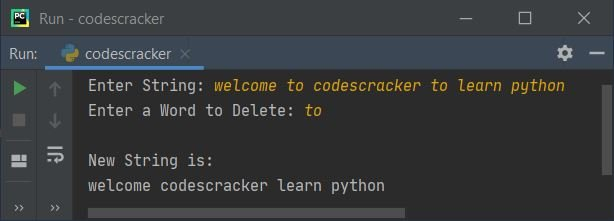From above program, the following statement:

`newtext = [x for x in wordlist if x not in word]`

is the short form of following block of code:

```newtext = []
for x in wordlist:
if x not in word:
newtext.append(x)```

#### Modified Version of Previous Program:

This is the modified version of previous program. The join() method in this program used in a way that, the list newtext is converted into a string:

```print("Enter String: ", end="")
text = input()
print("Enter a Word to Delete: ", end="")
word = input()

wordlist = text.split()
if word in wordlist:
newtext = [x for x in wordlist if x not in word]
newtext = ' '.join(newtext)
print("\nNew string after removing \"" +(word)+ "\":")
print(newtext)
else:

Here is its sample run with same user input as of previous program's sample run: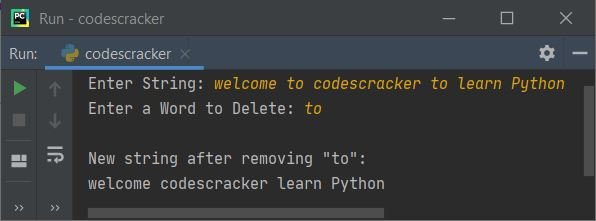#### Same Program in Other Languages

Python Online Test

« Previous Program Next Program »